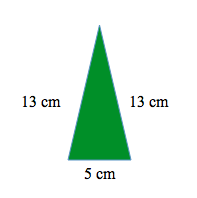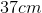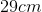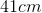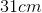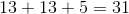ISEE Lower Level Quantitative : Triangles

Example Questions

← Previous 1 3 4 5 6 7 8 9 10 11

Example Question #1 : Triangles

The perimeter of a triangle is 14 inches.  One side is 4 inches long.  Another side is 5 inches long.  How long is the 3rd side?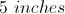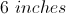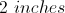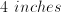Explanation:

Add up all of the sides of a triangle to get the perimeter.  Here, 2 of the sides add up to give you 9 inches.  The third side must equal the difference between 14 inches and 9 inches.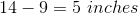Example Question #411 : Plane Geometry

Bill found the perimeter of the triangle below to be 34 units.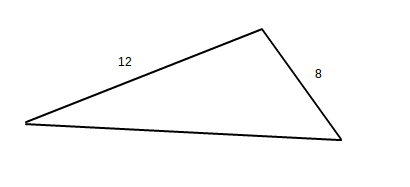What is the length of the missing side?

12 units

15 units

20 units

13 units

14 units

14 units

Explanation:

The perimeter of a triangle can be found by adding up all of the sides.

Start by adding the two sides that are given: 8 + 12 = 20

The third side can be found by subtracting this sum from the total perimeter: 34 - 20 = 14.  The missing side is 14 units long.

Example Question #1 : Triangles

Zoe drew the below triangle.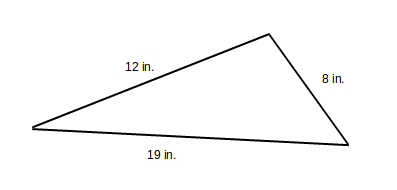What is the perimeter of the triangle?

36 inches

20 inches

40 inches

39 inches

30 inches

39 inches

Explanation:

The perimeter of a triangle can be found by adding all of the side lengths: 8 + 12 + 19 = P.  Therefore, the perimeter of the given triangle is 39 inches.

Example Question #3 : Triangles

Megan drew the triangle below and found its perimeter to be 36 inches.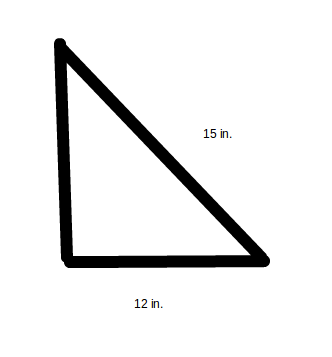What is the length of the missing side?

21 inches

36 inches

9 inches

15 inches

12 inches

9 inches

Explanation:

The perimeter of a triangle can be found by adding up all of the sides.

Start by adding the two sides that are given: 15 + 12 = 27

The third side can be found by subtracting this sum from the total perimeter: 36 - 27 = 9.  The missing side is 9 inches long.

Example Question #4 : Triangles

James found the perimeter of the triangle to be 95 cm.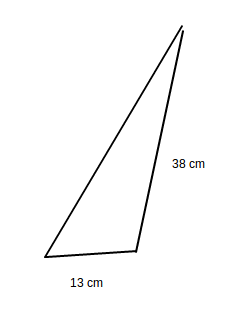What is the length of the missing side?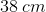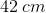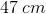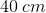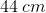Explanation:

To find the perimeter of a triangle, add the lengths of the three sides together. In the given triangle,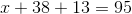. Combine like terms and solve forin order to find the length of the missing side: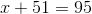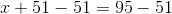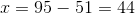The missing side islong.

Example Question #415 : Plane Geometry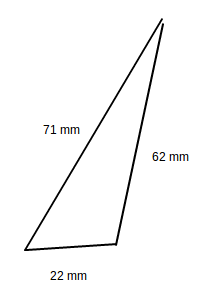What is the perimeter of the above triangle?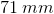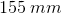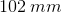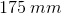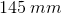Explanation:

To find the perimeter of a triangle, add up the lengths of the three sides: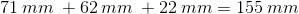Example Question #85 : Find Perimeter Or Missing Side Lengths Of Polygons: Ccss.Math.Content.3.Md.D.8

What is the perimeter of the triangle below?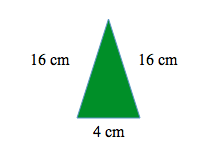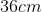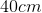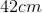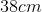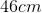Explanation:

To find the perimeter, we add all of the side lengths together.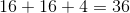Example Question #86 : Find Perimeter Or Missing Side Lengths Of Polygons: Ccss.Math.Content.3.Md.D.8

What is the perimeter of the triangle below?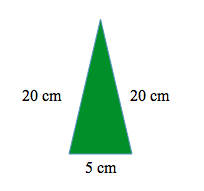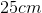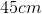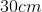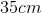Explanation:

To find the perimeter, we add all of the side lengths together.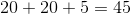Example Question #87 : Find Perimeter Or Missing Side Lengths Of Polygons: Ccss.Math.Content.3.Md.D.8

What is the perimeter of the triangle below?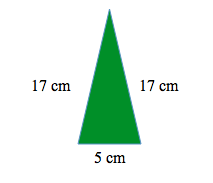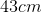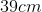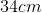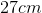Explanation:

To find the perimeter, we add all of the side lengths together.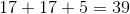Example Question #88 : Find Perimeter Or Missing Side Lengths Of Polygons: Ccss.Math.Content.3.Md.D.8

What is the perimeter of the triangle below?